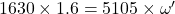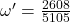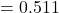## A 5.0-m radius playground merry-go-round with a moment of inertia of 1,630 kg m2 is rotating freely with an angular speed of 1.6 rad/s. Two

Question

A 5.0-m radius playground merry-go-round with a moment of inertia of 1,630 kg m2 is rotating freely with an angular speed of 1.6 rad/s. Two people, each having a mass of 69.5 kg, are standing right outside the edge of the merry-go-round and step on it with negligible speed. What is the angular speed of the merry-go-round right after the two people have stepped on

in progress 0
2 months 2021-07-30T08:20:00+00:00 1 Answers 8 views 0

The right solution is “0.511“.

Explanation:

Given:

Initial moment of inertia,

= 1630 kg.m²

= 5 m

Angular speed,

Now,

The moment of inertia after stepping on will be:

=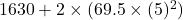=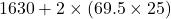=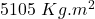hence,

As per the question, the angular speed is conserved, then